# Axisymmetric Peristaltic Flow of a Non-Newtonian Fluid in a Channel with Elastic Walls

Axisymmetric Peristaltic Flow of a Non-Newtonian Fluid in a Channel with Elastic Walls

Gangarapu Yasodhara Sreedharamalle Sreenadh Baina Sumalatha Akkiraju N.S. Srinivas

Department of Mathematics, Government Junior College for Girls, Chandragiri, Chittoor, 517 101, A.P., India

Department of Mathematics, Sri Venkateswara University, Tirupati 517 502, A.P., India

Department of Mathematics, Sri Venkateswara College of Engineering and Technology, Karakambadi Road, Tirupati 517 502, A.P., India

Department of Mathematics, School of Advanced Sciences, VIT, Vellore 632 014, T.N., India

Corresponding Author Email:
anssrinivas@vit.ac.in
Page:
315-323
|
DOI:
https://doi.org/10.18280/mmep.070219
20 March 2020
|
Accepted:
16 May 2020
|
Published:
30 June 2020
| Citation

OPEN ACCESS

Abstract:

This article explores the theoretical investigation of peristaltic motion of a non-Newtonian fluid accompanied in a horizontal channel with elastic walls. Most of the physiological fluids (blood) behaves like a non-Newtonian fluid. To characterize the fluid flow behavior, Casson fluid model is considered which a yield stress model and it holds good to explain the behavior of blood flow through small diameter conduits at low shear rates. The deformation in the walls of the channel is studied under two aspects, one is peristalsis and another is elasticity. Exact solutions are obtained for velocity and stream function. The size of the trapped food bolus increases with increasing values of yield stress parameter. The theoretical obtained results may be useful in understanding of pathological conditions arising due to change in elasticity and different peristaltic wave forms.

Keywords:

Casson fluid, peristaltic transport, elasticity, yield stress

1. Introduction

Peristaltic transport of biofluid includes movement of chyme in the gastrointestinal tract, urine passage from kidney to bladder, blood circulation, and locomotion of worms, ovum movement in the fallopian tube and spermatozoa in ductus efferents. Industrial applications of peristalsis may include transport of sanitary, corrosive and noxious materials. Such activity is also quite important in processes of pharmaceutical, cosmetic and paper industries. In view of this, Latham , Burns and Parkes  and Shapiro et al.  made earliest investigations of viscous fluid in a channel with peristaltic pumping. In present analysis, Casson model is considered which is a yield stress model and is used to explain the behavior of blood flow through small blood vessels at low shear rates. Merrill et al.  established that Casson model holds good for blood flow in small diameter conduits with certain wall shear stress limits. Afterwards the peristaltic movement for Newtonian and non-Newtonian fluids has been analyzed widely. Relevant information on the topic in view of diverse aspects is extensive. However, few most recent attempts in this regard can be consulted through the references [5-13].

Most of the theoretical work available in literature deals with tubes and channels with rigid walls. Various models are being proposed to study the effect of elasticity on living organisms. According to the available information about peristalsis, maximum consideration in the previous works has been concentrated on the flow of biofluid flows in channels with rigid walls. Consideration of elasticity present in the walls is important since many biological ducts like gastrointestinal tract, blood vessels, arteries and fallopian tube are elastic in nature. In this point of view, an attempt is made to investigate the flow of non-Newtonian fluid through channel walls having elastic nature with the peristalsis. The significance of elasticity for the pulse wave generating from the heart was first recognized by Young . Afterwards, theoretical and experimental works on flow of Newtonian/ non-Newtonian fluids with elastic conduits are made by the researchers [15-22].

Most the real fluids are characterized as shear-thinning yield stress material like Casson liquid. Currently in food stuff processing the Casson fluid model is used. Also, this model has been utilized to model the rheological behavior of chocolate. Furthermore, various working materials e.g. molten plastics, artificial fibers, polymeric materials, foodstuffs, blood, slurries and synovial liquids which demonstrate characteristics non-Newtonian liquids. Liquids of such kinds having relationships in the form of shear-stress-strain which are materially dissimilar from the viscous model. Various non-Newtonian models require some form of amendment in the force constraints. In the category of these non-Newtonian materials, Casson material has diverse vital features. In polymer processing industry and in the field of biomechanics it has considerable demands. Sometimes the Casson material is found very better for rheological data when compared with general viscoelastic models for various fluids. In view of the above facts, various researchers [23-26] studied the flow of Casson liquid with different physical characteristics.

Absi  proposed a new relation between pressure and area which is useful for understanding the flow behavior in arterial vessels. Nahar et al.  investigated the influence of both Newtonian and non-Newtonian fluid flow with low and high shear thinning fluids through various deformations of the tube. Choudhari et al.  examined the peristaltic flow of Bingham fluid through elastic tube with porous wall under the effect of variable viscosity. Wang et al.  proposed a model of three dimensional viscous flow in a compliant artery containing an aneurysm by employing the immersed boundary-lattice Boltzmann-finite element method which allows to adequately account for the elastic deformation of both the blood vessel and aneurysm walls.

In this paper, peristaltic transport of non-Newtonian fluid in a planar channel with elastic walls is investigated. Here Casson fluid is considered as non-Newtonian fluid. The expressions for velocity, stream function, flux and pressure rise are determined. The resulting equations are solved analytically subjected to the proper boundary conditions. To the best of literature survey, no discussion is made on trapping limits by considering both peristalsis and elasticity of the wall. Trapping phenomena is discussed. The results of the present study are analyzed though graphs. The theoretical investigations are more appropriate to analyse the biological systems. Obtained results may be useful in understanding the behavior of peristaltic transport of a blood flow in small blood vessels and different non-Newtonian yield stress fluids.

2. Problem Formulation

Figure 1 signifies the two-dimensional peristaltic pumping of an incompressible Casson fluid in a channel of length L and half width of the channel a(x) with flexible elastic walls. μ is the viscosity of the Casson fluid. The wall deformation of the channel is represented by the equation

$A(X, t)=a_{0}+b \sin \frac{2 \pi}{\lambda}(X-c t)$  (1)

Here the radius of the tube in the absence of elasticity is a0.

## 1.png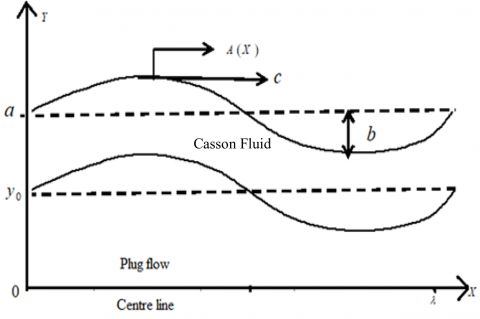Figure 1. Physical model

To study the problem we have transformed the stationary coordinates (X,Y) to moving coordinates (x,y) with the following transformations:

$u \rightarrow U-c, v \rightarrow V, x \rightarrow X-c t, y \rightarrow Y, p(x) \rightarrow P(X, t)$  (2)

After using the transformations given in Eq. (2) the governing equations of motion in the moving frame can be written as

$-\frac{\partial p}{\partial x}=\frac{\partial}{\partial y}\left(\tau_{y x}\right)$  (3)

where,

$\left(\tau_{y x}\right)^{\frac{1}{2}}=\left\{\begin{array}{ll}\mu^{\frac{1}{2}}\left(-\frac{\partial u}{\partial y}\right)^{\frac{1}{2}}+\tau_{0}^{\frac{1}{2}}, & \tau_{y x} \geq \tau_{0} \\ 0, & \tau_{y x}<\tau_{0}\end{array}\right.$   (4)

here the axial velocity is u, the yield stress parameter is $\tau$0, the shear stress is $\tau$yx and pressure is P.

Appropriate boundary conditions are

at $y=A(x) \quad : u=\frac{\partial \psi}{\partial y}=-c$  (5a)

at $y=0 \quad : u_{y}=\frac{\partial^{2} \psi}{\partial y^{2}}=0$  (5b)

at $y=a(x) : \psi=q$  (5c)

at $\mathrm{at} \cdot y=0 : \quad \psi=0$  (5d)

at $\cdot y=0 : \quad \tau_{y x}=0$ (5e)

Here, the stream function is represented by ψ.

3. Solution of the Problem

Eqns. (3) and (4) can be solved subject to the boundary conditions in (5) by using the following non-dimensional quantities:

$\bar{u}=\frac{u}{c} ; \bar{x}=\frac{x}{\lambda} ; \bar{y}=\frac{y}{a_{0}} ; \bar{a}=\frac{A(x)}{a_{0}} ; \bar{p}=\frac{p a_{0}^{2}}{\lambda \mu c} ; \bar{\psi}=\frac{\psi}{a_{0}}$

$\bar{q}=\frac{q}{a_{0} c} ; \bar{t}=\frac{c t}{\lambda} ; \phi=\frac{b}{a_{0}} ; \bar{\tau}_{0}=\frac{\tau_{0}}{\mu\left(c / a_{0}\right)} ; \bar{\tau}_{y x}=\frac{\tau_{y x}}{\mu\left(c / a_{0}\right)}$  (6)

Using the non-dimensional quantities given in Eq. (6), the momentum equation becomes (removing the bars)

$-\frac{\partial p}{\partial x}=\frac{\partial}{\partial y}\left(\tau_{y x}\right)$   (7)

here $\left(\tau_{y x}\right)^{\frac{1}{2}}=\left\{\begin{array}{ll}\left(-\frac{\partial u}{\partial y}\right)^{\frac{1}{2}}+\tau_{0}^{\frac{1}{2}}, & \tau_{y x} \geq \tau_{0} \\ 0, & \tau_{y x}<\tau_{0}\end{array}\right.$   (8)

The dimensionless boundary conditions are as follows:

at $y=a(x)=1+\phi \sin 2 \pi x \quad : u=\frac{\partial \psi}{\partial y}=-1$  (9a)

at $y=0 \quad$, $u_{y}=\frac{\partial^{2} \psi}{\partial y^{2}}=0$  (9b)

at $y=a(x) : \psi=q$  (9c)

at $y=0 : \psi=0$  (9d)

at $y=0 : \tau_{y x}=0$  (9e)

Eq. (7) can be solved by using the boundary conditions given in Eq. (9), one can obtain the velocity as

$u=-1+\frac{P}{2}\left(a^{2}-y^{2}\right)+\tau_{0}(a-y)+\frac{4}{3} P^{\frac{1}{2}} \tau_{0}^{\frac{1}{2}}\left(y^{\frac{3}{2}}-a^{\frac{3}{2}}\right)$  (10)

The upper limit of the plug flow region can be find by using the boundary condition $\frac{\partial u}{\partial y}=0$  at y=y0. But  $y_{0}=\frac{\tau_{0}}{P}$ . Also by using the condition $\tau$yx=$\tau$a at y=a, we get $P=\frac{\tau_{a}}{a}$ .

Therefore $\frac{y_{0}}{a}=\frac{\tau_{0}}{\tau_{a}}=\tau, \quad 0<\tau<1$  (11)

The velocity in the plug flow region is (put y=y0 in Eq. (10)),

$u_{p}=-1+\frac{P}{2}\left\{a^{2}+2 y_{0} a-\frac{1}{3} y_{0}^{2}-\frac{8}{3} y_{0}^{\frac{1}{2}} a^{\frac{3}{2}}\right\}$ (12)

Integrating Eqns. (10) and (12) and using the conditions ψ=0 at y=0 and ψ=ψp at y=y0, the stream function as

$\psi=-y+\frac{P}{2}\left\{\begin{array}{c}a^{2} y-\frac{y^{3}}{3}+2 y_{0}\left(a y-\frac{y^{2}}{2}\right) \\ +\frac{8}{3} y_{0}^{\frac{1}{2}}\left(\frac{2}{5} y^{\frac{5}{2}}-a^{\frac{3}{2}} y\right)\end{array}\right\}$

for $y_{0} \leq y \leq h$  (13)

The stream function in plug flow region as

$\psi_{p}=-y_{0}+\frac{P}{2}\left\{a^{2} y_{0}-\frac{4}{15} y_{0}^{3}+2 a y_{0}^{2}-\frac{8}{3} a^{\frac{3}{2}} y_{0}^{\frac{3}{2}}\right\}$  (14)

for 0≤yy0.

The flux q through in any cross section is

$q=\int_{0}^{y_{0}} u_{p} d y+\int_{y_{0}}^{a} u d y=-a+F \frac{P a^{3}}{3}$  (15)

$G=F \frac{P a^{3}}{3}$  (16)

where, $G=q+a ; F=1+\frac{3}{2} \tau-\frac{12}{5} \tau^{\frac{1}{2}}-\frac{1}{10} \tau^{3}$

The above Eq. (15) represents the volume flow rate of Casson fluid flow in a channel with peristalsis in the absence of elasticity.

Now the elasticity of the channel wall is taken in to consideration along with the peristalsis to determine the variation of flux.

Due to the pressure difference between the outside of the walls and fluid, there is a corresponding change in width of the channel a(x). From this, the flow follows famous Poiseuille law which states the flux is expressed as a linear function of pressure difference between inlet and outlet of the channel.

The flux and the pressure gradient are related by the expression 

$G=\sigma\left(p-p_{0}\right)\left(-\frac{d p}{d x}\right)$  (17)

where $\sigma\left(p-p_{0}\right)=F \frac{\left(a+a^{\prime}\right)^{3}}{3}$  (18)

Here σ is conductivity of the tube and a’ is a function of the pressure difference p(x)-p0, p0 is the pressure outside the tube and a denotes the wall movement due to peristalsis is a(x)=1+$\phi$sin2πx.

The equation (17) is integrating w.r.t. x from x=0 and using condition at the inlet p1=p(0), one can get

$\int_{0}^{1}(q+a) d x=\int_{p(x)-p_{0}}^{p_{1}-p_{0}} \sigma\left(p^{\prime}\right) d p^{\prime}$  (19)

where, p’=p(x)-p0.

To find flux q, set x=1 and p2=p(1), which yields

$\int_{0}^{1}(q+a) d x=\int_{p_{2}-p_{0}}^{p_{1}-p_{0}} \sigma\left(p^{\prime}\right) d p^{\prime}$  (20)

$q=-1+\frac{F}{3} \int_{p_{2}-p_{0}}^{p_{1}-p_{0}}\left(a^{3}+3 a^{2} a^{\prime}+3 a a^{\prime 2}+a^{\prime 3}\right) d p^{\prime}$  (21)

If the tension in the walls of the channel T(a’) is known as a function of a’, then a’(p’) can be obtained using the equilibrium position,

$\frac{T\left(a^{\prime}\right)}{a^{\prime}}=p-p_{0}$  (22)

Roach and Burton  determined the static pressure-volume relation which is converted into a tension versus length curve. Rubinow and Keller  gave the following equation by using least square method:

$T\left(a^{\prime}\right)=t_{1}\left(a^{\prime}-1\right)+t_{2}\left(a^{\prime}-1\right)^{5}$  (23)

where, t1=13 and t2=300.

From Eqns. (22) and (23), we get

$d p^{\prime}=\left\{\frac{t_{1}}{a^{\prime 2}}+t_{2}\left(4 a^{\prime 3}-15 a^{\prime 2}+20 a^{\prime}-10+\frac{1}{a^{\prime 2}}\right)\right\} d a^{\prime}$  (24)

Substituting the Eq. (24) in (21), evaluated the integral by using Matlab package, and the flux of the fluid is given by

$\left.\begin{array}{l}q+1= \\ \frac{F}{3} \int_{\mathrm{p}_{2}-p_{0}}^{\mathrm{p}_{1}-p_{0}}\left[\left(a^{3}+3 a^{2} a^{\prime}+3 a a^{\prime 2}+a^{\prime 3}\right)\right. \\ \left(\frac{t_{1}}{a^{\prime 2}}+t_{2}\left(4 a^{\prime 3}-15 a^{\prime 2}+20 a^{\prime}-10+\frac{1}{a^{\prime 2}}\right)\right)\end{array}\right\} d a^{\prime}$  (25)

$q=\frac{F}{3}\left[g\left(a_{1}^{\prime}\right)-g\left(a_{2}^{\prime}\right)\right]-1$  (26)

where,

$g\left(a^{\prime}\right)=\left\{\begin{array}{l}a^{3}\left[\frac{-t_{1}}{a^{\prime}}+t_{2}\left(a^{\prime 4}-5 a^{\prime 3}+10 a^{\prime 2}-10 a^{\prime}-\frac{1}{a^{\prime}}\right)\right] \\ +3 a^{2}\left[t_{1} \log a^{\prime}+t_{2}\left(\begin{array}{c}\frac{4}{5} a^{\prime 5}-\frac{15}{4} a^{\prime 4}+\frac{20}{3} a^{\prime 3} \\ -5 a^{\prime 2}+\log \left(a^{\prime}\right)\end{array}\right)\right] \\ +3 a\left[t_{1} a^{\prime}+t_{2}\left(\frac{2}{3} a^{\prime 6}-3 a^{\prime 5}+5 a^{\prime 4}-\frac{10}{3} a^{\prime 3}+a^{\prime}\right)\right] \\ +\frac{t_{1}}{2} a^{\prime 2}+t_{2}\left(\frac{4}{7} a^{\prime 7}-\frac{5}{2} a^{\prime 6}+4 a^{\prime 5}-\frac{5}{2} a^{\prime 4}+a^{\prime 2}\right)\end{array}\right\}$; $a_{1}^{\prime}=a_{1}^{\prime}\left(p_{1}-p_{0}\right) ; a_{2}^{\prime}=a_{2}^{\prime}\left(p_{2}-p_{0}\right)$                 (27)

The pressure rise per wavelength for Casson fluid throug a channel with elastic walls with peristalsis is calculated using the Eqns. (13) and (14) is

$\Delta p=-\int_{0}^{1} \frac{3(\Theta-1+a)}{F\left(a+a^{\prime}\right)^{3}} d x$  (28)

where, Θ is the time averaged volumetric flow rate defined as

$\Theta=\frac{1}{T} \int_{0}^{T} Q d t=q+1$  (29)

Here, Q is the volumetric flow rate in stationary frame defined as

$Q=\int_{0}^{a}(u+1) d y=q+a$  (30)

4. Discussion of the Results

For the sake of conclusion of the behavior of dimensionless velocity field, flux and pressure rise and to get some tangible perception of the obtained solutions, successive numerical calculations were accomplished for different values of pertinent constraints like elastic parameters t1&t2, amplitude ratio $\phi$ and yield stress parameter $\tau$.

From Eq. (26), flux is calculated as a function of width of the channel. Figures 2-6 illustrate the effect of existing parameters on the flux with respect to width of the channel. From Figures 2 and 3, it is depicted that the flux is an increasing function of t1&t2. Figure 4 shows the profiles of flux for different values of $\phi$ which states that flux is increasing with increasing values of $\phi$ . In Figure 5, consequences of yield stress upon flux profiles are shown. This indicates that flux of the fluid is decreasing with increasing yield stress parameter. In Figure 6, flux profiles for different peristaltic wave forms are discussed. More flux is observed in the case square wave when compared to sinusoidal and Triangular wave.

Eq. (26) shows that the flux is a function of axial coordinate x. Figures 7-12 illustrate the effect of t1, t2, $\phi$ , $\tau$, a1’ and a2 on flux with respect to the axial coordinate x . The effect of elastic parameters t1&t2 on flux are depicted in Figures 7 and 8. It is observed that as the elastic parameters increases, flux remarkably increases. Figure 9 exemplifies the effect of amplitude ration $\phi$ on flux. It is observed that the flux increases with increasing values of $\phi$ . The effect of yield stress on flux is shown in Figure 10. It is worthwhile to note the flux is a decreasing function of yield stress. Figures 11 and 12 demonstrates the influence of inlet and outlet width of the channel a1’ and a2’ respectively on flux of the Casson fluid. It is observed that the flux profile decreases as the value of the a1’ increases. Opposite behavior is noticed in the case of a2’.

## 2.png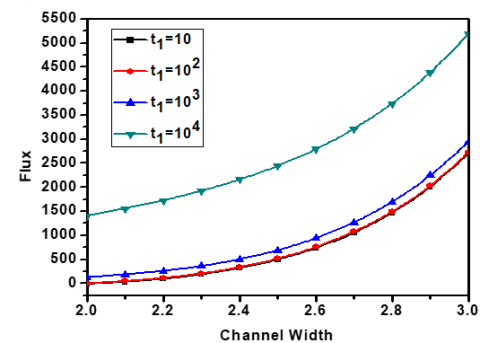Figure 2. Flux vs. width of the channel for different t1 with fixed values of $\tau$=0.6, t2=300, f=0.6, x=0.1

## 3.png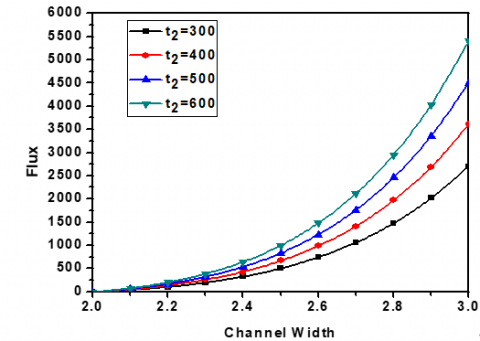Figure 3. Flux vs. width of the channel for different t2 with fixed values of $\tau$=0.6, t1=13, $\phi$=0.6, x=0.1

## 4.pngFigure 4. Flux vs. width of the channel for different $\phi$ with fixed values of t2=300, t1=13, $\tau$=0.6, x=0.1

## 5.png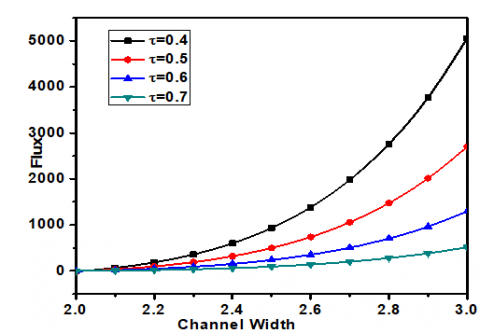Figure 5. Flux vs. width of the channel for different $\tau$ with fixed values of t2=300, t1=13, $\phi$=0.6, x=0.1

## 6.png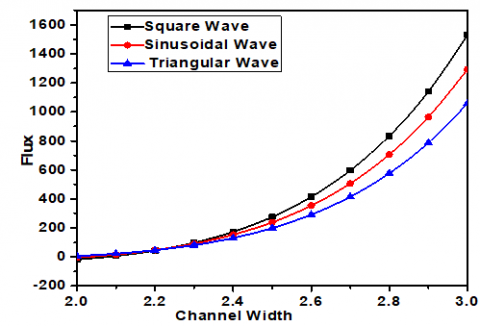Figure 6. Flux vs. width of the channel for different wave forms with fixed values of  $t_{2}=300, t_{1}=13, \phi=0.6, x=0.1, \beta=2$

## 7.png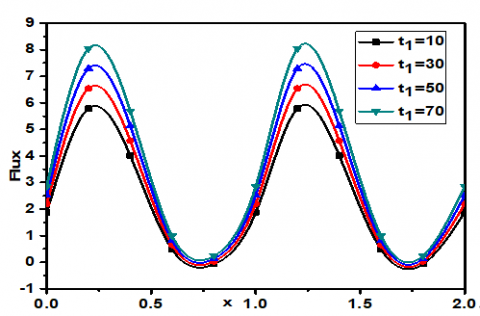Figure 7. Flux vs. x for different t1 with fixed values of  $\beta=2, t_{2}=300, \phi=0.4, a_{1}^{\prime}=0.2, a_{2}^{\prime}=0.3$

## 8.png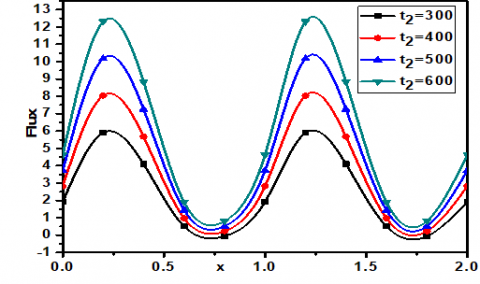Figure 8. Flux vs. x for different t2 with fixed values of  $\beta=2, t_{1}=13, \phi=0.4, a_{1}^{\prime}=0.2, a_{2}^{\prime}=0.3$

## 9.png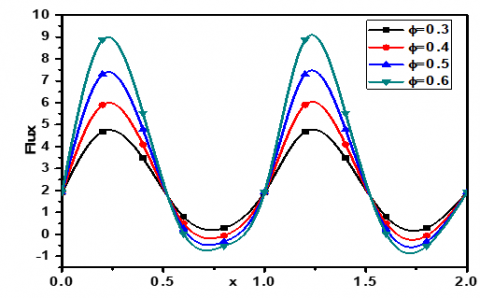Figure 9. Flux vs. x for different $\phi$ with fixed values of $t_{2}=300, t_{1}=13, \beta=2, a_{1}^{\prime}=0.2, a_{2}^{\prime}=0.3$

## 10.png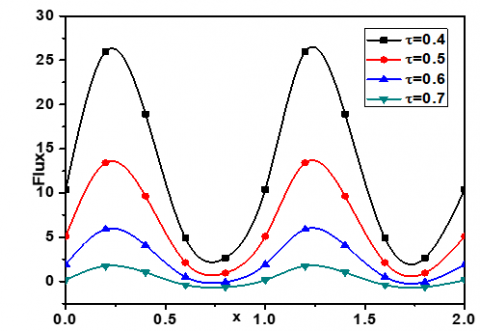Figure 10. Flux vs.x for different $\tau$ with fixed fixed values of  $t_{2}=300, t_{1}=13, \phi=0.4, a_{1}^{\prime}=0.2, a_{2}^{\prime}=0.3$

## 11.pngFigure 11. Flux vs.x for different  a1’ with fixed values of $t_{2}=300, t_{1}=13, \tau=0.6, \phi=0.4, a_{2}^{\prime}=0.3$

## 12.png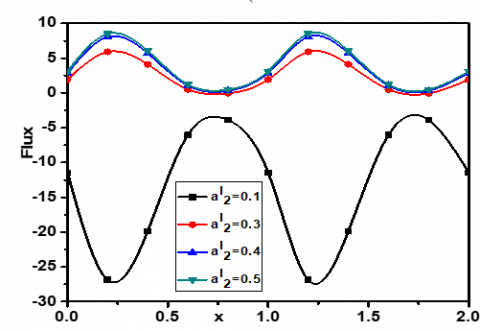Figure 12. Flux vs.x for different  a1’with fixed values of  $t_{2}=300, t_{1}=13, \tau=0.6, \phi=0.4, a_{1}^{\prime}=0.2$

## 13.png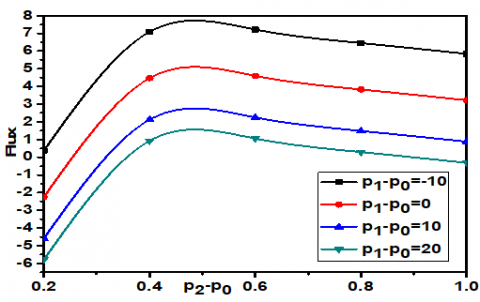Figure 13. Flux vs.p2-p0 for different p1-p0 with fixed values of t2=300, t1=13, $\phi$=0.6, $\phi$=0.4

## 14.pngFigure 14. Flux vs. p1-p0 for different p2-p0 with fixed values of t2=300, t1=13, $\phi$=0.6,$\phi$f=0.4

## 15.png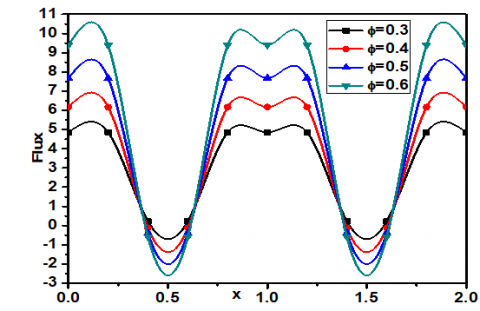Figure 15. Flux vs. x for different $\phi$ with fixed values of $t_{2}=300, t_{1}=13, \tau=0.6, a_{1}^{\prime}=0.2, a_{2}^{\prime}=0.3$  (square wave)

## 16.png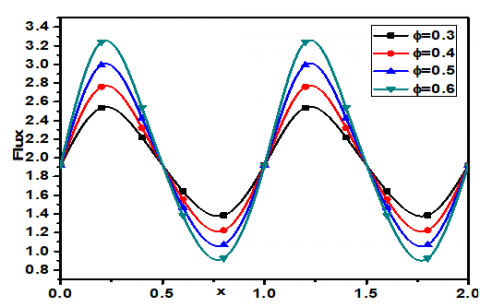Figure 16. Flux vs. x for different $\phi$ with fixed values $t_{2}=300, t_{1}=13, \tau=0.6, a_{1}^{\prime}=0.2, a_{2}^{\prime}=0.3$ (Triangular wave)

## 17.pngFigure 17. $\Delta p$ vs. Θ for different $\phi$ with fixed values of $\tau=0.6, a_{1}^{\prime}=0.25$

## 18.png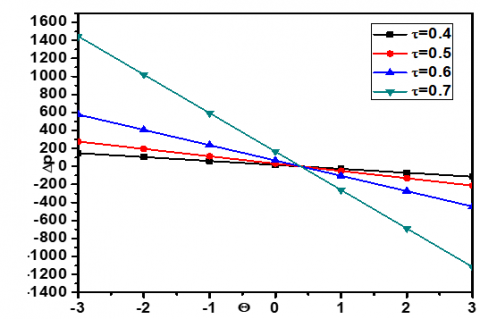Figure 18. $\Delta p$ vs. Θ for different $\tau$ with fixed values of a’=0.25, $\phi$=0.4

## 19.png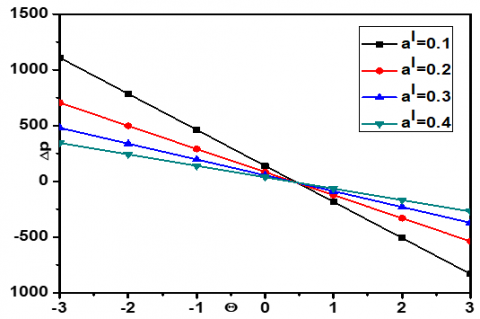Figure 19. $\Delta p$ vs. Θ for different a’ with fixed values of $\tau$=0.6, $\phi$=0.4

Figures 13 and 14 respectively, shows the variations of flux with the outlet for different values of inlet pressure and variations in flux with inlet pressure for different values of outlet pressure. Flux decreases with increasing values of inlet pressure for a fixed outlet pressure. Opposite behavior is noticed in the case of increasing values of outlet pressure. This phenomenon exists due to the mechanism of peristaltic pumping. Figures 15 and 16 show the effect of $\phi$ on the flux with respect to axial distance x for two different peristaltic wave forms namely square wave and triangular wave. More flux is observed in the case of square wave.

From Eq. (28), pressure rise $\Delta p$ is expressed as a function of time averaged flow rate Θ. Figures 17-18 show the effect of $\phi$,$\tau$,a’ on pressure rise. From Figures 17 and 18, it can be clearly seen that for a given Θ, $\Delta p$ depends upon $\phi$, $\tau$ and it increases with increasing $\phi$, $\tau$ respectively. $\Delta p$ increases with the increasing values of a’ which is evident from Figure 19.

5. Trapping Phenomena

Trapping is another important phenomenon observed in peristaltic transport. By analysis, one can gets the trapping limits when Θmin<Θ<Θmax.

where,

$\Theta_{\min }=-\phi+\frac{2 T\left(1+\phi+a^{\prime}\right)^{3}}{3(1+\phi)^{2}}$

and  $\Theta_{\mathrm{max}}=\phi+\frac{2 T\left(1-\phi+a^{\prime}\right)^{3}}{3(1-\phi)^{2}}$  (31)

Here $T=\frac{1+\frac{3}{2} \tau-\frac{12}{5} \tau^{\frac{1}{2}}-\frac{1}{10} \tau^{3}}{1-\frac{4}{15} \tau^{2}+2 \tau-\frac{8}{3} \tau^{\frac{1}{2}}}$

Special cases:

1. When a’=0 and $\tau$=0 from Eq. (31), we obtain the trapping limits for the peristaltic transport of a Newtonian fluid in the absence of elasticity as $\frac{2-\phi}{3}<\Theta<\frac{2+\phi}{3}$  which agrees with the results of Jaffrin and Shapiro .
2. When a’=0 form equation (31), we obtain the trapping limits for the peristaltic transport of a Casson fluid in the absence of elasticity as $-\phi+\frac{2 T}{3}(1+\phi)<\Theta<\phi+\frac{2 T}{3}(1-\phi)$.
3. When $\tau$=0 form equation (31), we obtain the trapping limits for the peristaltic transport of a Newtonian fluid in the presence of elasticity as $-\phi+\frac{2(1+\phi+a)^{3}}{3(1+\phi)^{2}}<\Theta<\phi+\frac{2\left(1-\phi+a^{\prime}\right)^{3}}{3(1-\phi)^{2}}$

In Figure 20, the streamlines for the trapping phenomenon are presented for Casson fluid flow with and without elasticity effects and Newtonian fluid flow with and without elasticity effects with Θ=0.6, =0.5 and a’=0.1. From the Figures of Casson fluid and Newtonian fluid with elasticity effect, we observed that effect of yield stress is to increase the size of the trapped bolus. From the Figures of Casson fluid flow with and without elasticity effect and Newtonian fluid flow with and without elasticity effect, it is noticed that the effect of elasticity is to decrease the size of the trapped bolus.

## a.png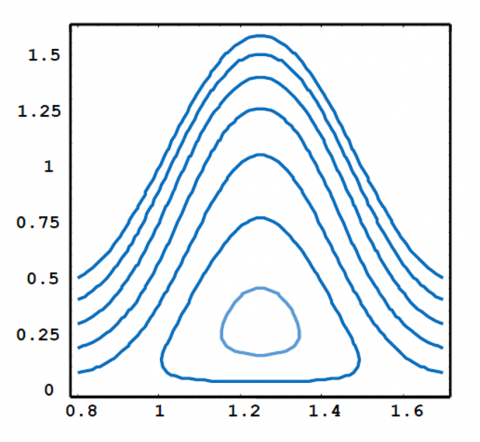(a) Trapping for Casson fluid with $\tau$=0.5, a’=0.1, $\phi$=0.5, Θ=0.6 (Channel with elastic walls)

## b.png(b) Trapping for Casson fluid with$\tau$=0.5, a’=0, $\phi$=0.5, Θ=0.6 (Channel with inelastic walls)

## c.png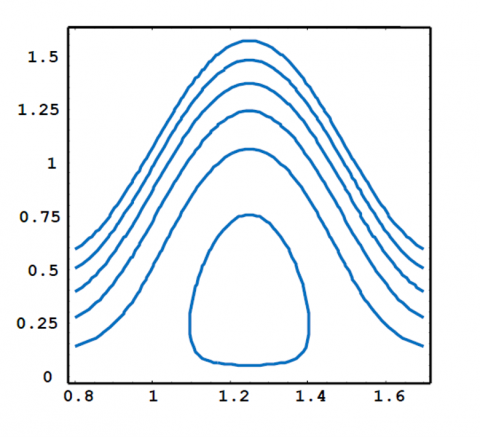(c) Trapping for Newtonian fluid with $\tau$=0, a’=0, $\phi$=0.5, Θ=0.6 (Channel with inelastic walls)

## d.png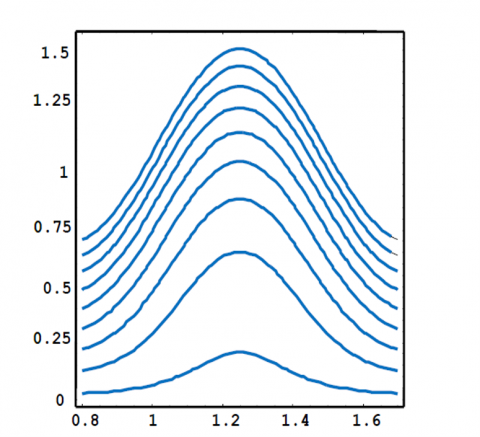(d) Trapping for Newtonian fluid with $\tau$=0, a’=0.1, $\phi$=0.5, Θ=0.6 (Channel with elastic walls)

Figure 20. Trapping phenomena

The non-dimensional expressions for the three different wave forms are expressed (i) Sinusoidal wave (ii) Triangular wave (iii) Square wave respectively

$a(x)=1+\phi\left\{\begin{array}{l}\sin 2 \pi x-----(\mathrm{i}) \\ {\left[\frac{32}{\pi^{2}} \sum_{m=1}^{\infty} \frac{\sin \frac{\pi}{8}(2 m-1)}{(2 m-1)^{2}} \sin [(2 m-1) \pi x]\right]-(\mathrm{i} \mathrm{i})} \\ {\left[\frac{4}{\pi} \sum_{m=1}^{\infty} \frac{(-1)^{m+1}}{(2 m-1)} \cos [(2 m-1) \pi x]\right]---(\mathrm{iii})}\end{array}\right.$

6. Conclusions

The present article devoted to investigate the peristaltic movement of a non-Newtonian fluid through a channel with elastic walls. Biofluid (blood) is modeled as a Casson fluid. It is used to study the changes in the blood flow pattern. The flow is examined under the consideration of yield stress parameter along with the effects of wall elasticity and peristalsis. Different peristatic wave forms are taken into consideration. The governing equations are solved analytically. The results are analyzed for different physical parameter through graphs. On the analysis of the present study, we conclude that the flux increases with increasing of elastic parameters. Enhancing the values of yield stress parameters the flux decreases. One can observe that more flux is observed in the case of square wave than sinusoidal wave and triangular wave. Pressure rise increases with the increasing of amplitude ratio and pressure rise increases with increasing yield stress parameter. The trapped bolus size is increases with increasing values of yield stress parameter. The present study explores the blood flow in pathological conditions. Furthermore, the results obtained from the present study will be useful in validating the results of more complex theoretical studies to be carried out in future.

Acknowledgement

The authors express sincere thanks to the referees for their valuable suggestions in the improvement of the paper.

References

 Latham, T.W. (1966). Fluid motion in a peristaltic pump. Doctoral dissertation, Massachusetts Institute of Technology.

 Burns, J.C., Parkes, T. (1967). Peristaltic motion. Journal of Fluid Mechanics, 29(4): 731-743. https://doi.org/10.1017/S0022112067001156

 Shapiro, A.H., Jaffrin, M.Y., Weinberg, S.L. (1969). Peristaltic pumping with long wavelengths at low Reynolds number. Journal of Fluid Mechanics, 37(4): 799-825. https://doi.org/10.1017/S0022112069000899

 Merrill, F.W., Benis, A.M., Gilliland, E.R., Sherwood, T.K., Salzman, E.W. (1965). Pressure-flow relations of human blood in hollow fibers at low flow rates. Journal of Applied Physiology, 20(5): 954-967. https://doi.org/10.1152/jappl.1965.20.5.954

 Jaffrin, M.Y., Shapiro, A.H. (1971). Peristaltic pumping. Annual Review of Fluid Mechanics, 3(1): 13-37. https://doi.org/10.1146/annurev.fl.03.010171.000305

 Bugliarello, G., Sevilla, J. (1970). Velocity distribution and other characteristics of steady and pulsatile blood flow in fine glass tubes. Biorheology, 7(2): 85-107. https://doi.org/10.3233/bir-1970-7202

 Shukla, J.B., Parihar, R.S., Rao, B.R.P., Gupta, S.P. (1980). Effects of peripheral-layer viscosity on peristaltic transport of a biofluid. Journal of Fluid Mechanics, 97(2): 225-237. https://doi.org/10.1017/ S0022112080002534

 Radhakrishnamacharya, G. (1982). Long wavelength approximation to peristaltic motion of a Power-law fluid. Rheologica Acta, 21: 30-35. https://doi.org/10.1007/BF01520703

 Brasseur, J.G., Corrsin, S. (1987). The influence of a peripheral layer of different viscosity on peristaltic pumping with Newtonian fluids. Journal of Fluid Mechanics, 174: 495-519. https://doi.org/10.1017/S0022112087000211

 Vajravelu, K., Sreenadh, S., Rajanikanth, K., Lee C. (2012). Peristaltic transport of a Williamson fluid in asymmetric channels with permeable walls. Nonlinear Analysis: Real World Applications, 13(6): 2804-2822. https://doi.org/10.1016/j.nonrwa.2012.04.008

 Rao, A.R., Mishra, M. (2004). Peristaltic transport of a power-law fluid in a porous tube. Journal of non-Newtonian Fluid Mechanics, 121: 163-174. https://doi.org/ 10.1016/j.jnnfm.2004.06.006

 Nadeem, S., Sadaf, H. (2016). Hypothetical analysis for peristaltic transport of metallic nanoparticles in an inclined annulus with variable viscosity. Bulletin of the Polish Academy of Sciences: Technical Sciences, 64(2): 447-454. https://doi.org/10.1515/bpasts-2016-0050

 Misra, J.C., Mallick, B., Sinha, A. (2018). Heat and mass transfer in asymmetric channels during peristaltic transport of an MHD fluid having temperature-dependent properties. Alexandria Engineering Journal, 57: 391-406. https://doi.org/10.1016/j.aej.2016.09.021

 Young, T. (1880). Hydraulic investigations, subservient to an intended Croonian lecture on the motion of the blood. Proceedings of the Royal Society of London, 8: 164-186. https://doi.org/10.1098/rstl.1808.0014

 Rubinow, S.I., Keller, J.B. (1972). Flow of a viscous fluid through an elastic tube with applications to blood flow. Journal of Theoretical Biology, 35: 299-313. https://doi.org/10.1016/0022-5193(72)90041-0

 Burton, A.C. (1951). On the physical equilibrium of small blood vessels. American Journal of Physiology-Legacy Content, 164(2): 319-329. https://doi.org/10.1152/ajplegacy.1951.164.2.319

 Roach, M.R., Burton, A.C. (1959). The effect of age on the elasticity of human iliac arteries. Canadian Journal of Biochemistry and Physiology, 37(4): 557-570. https://doi.org/10.1139/o59-059

 Vajravelu, K., Sreenadh, S., Devaki, P., Prasad, K.V. (2011). Mathematical model for a Herschel-Bulkley fluid flow in an elastic tube. Central European Journal of Physics, 9(5): 1357-1365. https://doi.org/10.2478/s11534-011-0034-3

 Vajravelu, K., Sreenadh, S., Devaki, P., Prasad, K.V. (2015). Peristaltic transport of a Herschel- Bulkley fluid in an elastic tube. Heat Transfer-Asian Research, 44(7): 585-598. https://doi.org/10.1002/htj.21137

 Vajravelu, K., Sreenadh, S., Devaki, P., Prasad, K.V. (2016). Peristaltic pumping of a Casson fluid in an elastic tube. Journal of Applied Fluid Mechanics, 9(4): 1897-1905. doi: 10.18869/acadpub.jafm.68.235.24695

 Malik, M.Y., Khan, M. Salahuddin, T., Khan, I. (2016). Variable viscosity and MHD flow in Casson fluid with Cattaneo-Christov heat flux model: Using Keller box method. Engineering Science Technology, an International Journal, 19: 1985-1992. https://doi.org/10.1016/j.jestch.2016.06.008

 Srinivas, A.N.S., Selvi, C.K., Sreenadh, S. (2017). Peristaltic pumping of a generalized Newtonian fluid in an elastic tube. Journal of Applied Fluid Mechanics, 10(6): 1785-1798. https://doi.org/10.29252/jafm.73.245.27908

 Khan, M.I., Waqas, M., Hayat, T., Alsaedi, A. (2017). A comparative study of Casson fluid with homogeneous-heterogeneous reactions. Journal of Colloid Interface Science, 498: 85-90. https://doi.org/10.1016/j.jcis.2017.03.024

 Tamoor, M., Waqas, M., Khan, M.I., Alsaedi, A., Hayat, T. (2017). Magnetohydrodynamic flow of Casson fluid over a stretching cylinder. Results in Physics, 7: 498-502. https://doi.org/10.1016/j.rinp.2017.01.005

 Aghighi, M.S., Ammar, A., Metivier, C., Gharagozlu, M. (2018). Rayleigh-Bénard convection of Casson fluids. International Journal of Thermal Sciences, 127: 79-90. https://doi.org/10.1016/j.ijthermalsci.2018.01.016

 Mahanthesh, B., Gireesha, B.J. (2018). Thermal Marangoni convection in two-phase flow of dusty Casson fluid. Results in Physics, 8: 537-544. https://doi.org/10.1016/j.rinp.2017.12.066

 Absi, R. (2018). Revisiting the pressure-area relation for the flow in elastic tubes: Application to arterial vessels. Series on Biomechanics, 32(1): 47-59. https://hal.archives-ouvertes.fr/hal-01807385

 Nahar, S., Dubey, B.N., Windhab, E.J. (2019). Influence of flowing fluid property through an elastic tube on various deformations along the tube length. Physics of Fluids, 31(10): 101905. https://doi.org/10.1063/1.5123182

 Choudhari, R., Gudekote, M., Vaidya, H., Prasad, K.V., Khan, S.U. (2020). Rheological effects on peristaltic transport of Bingham fluid through an elastic tube with variable fluid properties and porous walls. Heat Transfer. https://doi.org/10.1002/htj.21779

 Wang, H., Krüger, T., Varnik, F. (2020). Effects of size and elasticity on the relation between flow velocity and wall shear stress in side-wall aneurysms: A lattice Boltzmann-based computer simulation study. PLoS ONE, 15(1): e0227770. https://doi.org/10.1371/journal.pone.0227770